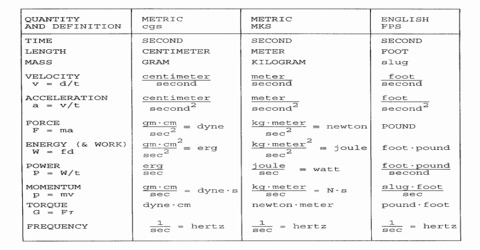# Procedure to Write Units

Procedure to Write Units

Some rules of writing unit as accepted in the international conference in 1960 are mentioned below:

(i) Unit is to be written in singular number. e.g., km but not kms.

(ii) A full stop symbol cannot be put at the end of the unit, e.g. km, but not km.

(iii) Rule of writing decimal is 1.9, but it is also written as 1·9.

(iv) In order to read a long number conveniently, three numbers around a decimal are written together. viz:

• Incorrect: 24765.321
• Correct 24,765·321

(v) While writing unit separation sign (/) e.g. (N/m2) can be used only once. But it is advised not to use it. For example instead of N/m2 one should write Nm-2.

(vi) Decimals of a unit should be written in the following way—

• deci ( = 10-1)d
• centi (= 10-2) c etc.

In normal unit minutes, hours, days, weeks, months, years etc are allowed but in real scientific measurement this type of unit should not be used.